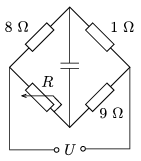Mathematical and Physical Journal
for High Schools
Issued by the MATFUND Foundation
 Already signed up? New to KöMaL?

#Problem P. 4534. (April 2013)

P. 4534. What is the value of the resistance of the variable resistor R shown in the figure, when

a) the condenser is uncharged;

b) the voltage across the condenser is exactly half of U?(4 pont)

Deadline expired on May 10, 2013.

Sorry, the solution is available only in Hungarian. Google translation

Megoldásvázlat. $\displaystyle a)$ $\displaystyle R=72~\Omega$.

$\displaystyle b)$ $\displaystyle R=\frac{63}{11}~\Omega\approx 5{,}7~\Omega$.

### Statistics:

 76 students sent a solution. 4 points: 60 students. 3 points: 11 students. 2 points: 4 students. 0 point: 1 student.

Problems in Physics of KöMaL, April 2013